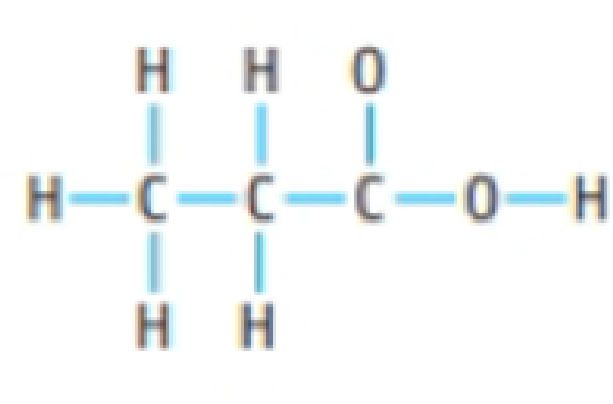Chapter 8, Problem 7PS

Chapter
Section
Textbook Problem

Draw a Lewis structure for each of the following molecules: (a) chlorodifluoromethane, CHClF2 (b) propanoic acid, C2H5CO2H (basic structure pictured below)(c) acetonitrile, CH3CN (the framework is H3C—C—N) (d) allene, H2CCCH2

(a)

Interpretation Introduction

Interpretation: It should be drawn the Lewis structure for the given following molecule or ions.

Concept Introduction:

• Lewis structures are diagrams that represent the chemical bonding of covalent molecules and coordination compounds.
• It is also known as Lewis dot structures which represent the bonding between atoms of a molecule and the lone pairs of electrons that may exist in the molecule.
• While drawing the skeletal structure, the least electronegative atom is considered as the central atom.
• If the more electronegative atom was placed as the central atom, then the concentration of negative charges becomes high which leads to the instability of the molecule.
Explanation

In CHClF3 molecule the number of valence electron is 26.

The Lewis structure for the given molecule is given as below:

(b)

Interpretation Introduction

Interpretation: It should be drawn the Lewis structure for the given following molecule or ions.

Concept Introduction:

• Lewis structures are diagrams that represent the chemical bonding of covalent molecules and coordination compounds.
• It is also known as Lewis dot structures which represent the bonding between atoms of a molecule and the lone pairs of electrons that may exist in the molecule.
• While drawing the skeletal structure, the least electronegative atom is considered as the central atom.
• If the more electronegative atom was placed as the central atom, then the concentration of negative charges becomes high which leads to the instability of the molecule.

(c)

Interpretation Introduction

Interpretation: It should be drawn the Lewis structure for the given following molecule or ions.

Concept Introduction:

• Lewis structures are diagrams that represent the chemical bonding of covalent molecules and coordination compounds.
• It is also known as Lewis dot structures which represent the bonding between atoms of a molecule and the lone pairs of electrons that may exist in the molecule.
• While drawing the skeletal structure, the least electronegative atom is considered as the central atom.
• If the more electronegative atom was placed as the central atom, then the concentration of negative charges becomes high which leads to the instability of the molecule.

(d)

Interpretation Introduction

Interpretation: It should be drawn the Lewis structure for the given following molecule or ions.

Concept Introduction:

• Lewis structures are diagrams that represent the chemical bonding of covalent molecules and coordination compounds.
• It is also known as Lewis dot structures which represent the bonding between atoms of a molecule and the lone pairs of electrons that may exist in the molecule.
• While drawing the skeletal structure, the least electronegative atom is considered as the central atom.
• If the more electronegative atom was placed as the central atom, then the concentration of negative charges becomes high which leads to the instability of the molecule.

Still sussing out bartleby?

Check out a sample textbook solution.

See a sample solution

The Solution to Your Study Problems

Bartleby provides explanations to thousands of textbook problems written by our experts, many with advanced degrees!

Get Started

Find more solutions based on key concepts